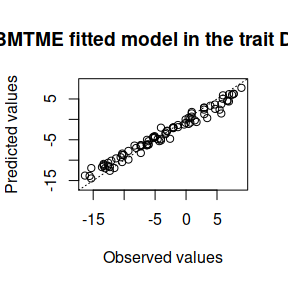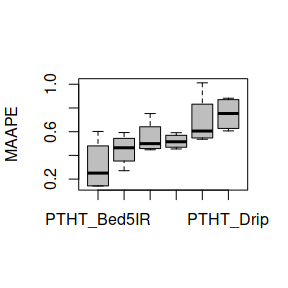# Fit the Bayesian Multi-Trait Multi-Environment model and apply a random partition cross-validation

#### 2019-02-11

This example illustrates how to fit a model with multiple environments and multiple traits using cross validation with random partitions. To do this, use the WheatToy data set to load, use the data() function as shown below:

library(BMTME)
data('WheatToy')

With this command, the following 2 objects are loaded into the R environment:

• genoWheatToy: Genomic matrix of the data set.
• phenoWheatToy: Phenotypic data from the dataset.

The phenotypic data has the following structure:

Gid Env DTHD PTHT
6861897 Bed5IR -10.327625 -14.3842610
6861897 Drip -4.054953 6.0095080
6861897 Bed2IR -7.307692 -0.0874643
6862028 Bed5IR -6.327625 -5.4364553
6862028 Drip -2.054953 6.5172227
6862028 Bed2IR -5.307692 4.4819052

Then the model to be adjusted must be defined, as the data set includes multiple environments and multiple traits, the BMTME model is used, and for its implementation, first we need order the dataset as follows:

phenoWheatToy <- phenoWheatToy[order(phenoWheatToy$Env, phenoWheatToy$Gid),]

We empathize do this, because if the dataset is not ordered by the environments and the identifiers, may cause conflicts between the analysis producing incorrect estimations. Then the matrix design for the genetic effects, the environment and the genotypic interaction per environment must be generated, as shown below,

LG <- cholesky(genoWheatToy)
ZG <- model.matrix(~0 + as.factor(phenoWheatToy$Gid)) Z.G <- ZG %*% LG Z.E <- model.matrix(~0 + as.factor(phenoWheatToy$Env))
ZEG <- model.matrix(~0 + as.factor(phenoWheatToy$Gid):as.factor(phenoWheatToy$Env))
G2 <- kronecker(diag(length(unique(phenoWheatToy$Env))), data.matrix(genoWheatToy)) LG2 <- cholesky(G2) Z.EG <- ZEG %*% LG2 Y <- as.matrix(phenoWheatToy[, -c(1, 2)]) Finally, the model is adjusted, with the commands provided below: fm <- BMTME(Y = Y, X = Z.E, Z1 = Z.G, Z2 = Z.EG, nIter = 1250, burnIn = 500, thin = 2, bs = 50, progressBar = FALSE) To know all the details about the results of the model fit using the BMTME function, we will use the str() function, which returns the following information: str(fm) #> List of 19 #>$ Y          : num [1:90, 1:2] -7.31 -5.31 5.69 6.69 -11.31 ...
#>   ..- attr(*, "dimnames")=List of 2
#>   .. ..$: chr [1:90] "3" "6" "9" "12" ... #> .. ..$ : chr [1:2] "DTHD" "PTHT"
#>  $nIter : num 1250 #>$ burnIn     : num 500
#>  $thin : num 2 #>$ dfe        : int 5
#>  $Se : num [1:2, 1:2] 160 -10.7 -10.7 157.8 #> ..- attr(*, "dimnames")=List of 2 #> .. ..$ : chr [1:2] "DTHD" "PTHT"
#>   .. ..$: chr [1:2] "DTHD" "PTHT" #>$ yHat       : num [1:90, 1:2] -8.25 -4.33 1.43 4.64 -9.57 ...
#>   ..- attr(*, "dimnames")=List of 2
#>   .. ..$: NULL #> .. ..$ : chr [1:2] "DTHD" "PTHT"
#>  $SD.yHat : num [1:90, 1:2] 2.24 2.68 2.61 2.7 2.65 ... #> ..- attr(*, "dimnames")=List of 2 #> .. ..$ : NULL
#>   .. ..$: chr [1:2] "DTHD" "PTHT" #>$ beta       : num [1:3, 1:2] -3.254 -5.199 -0.233 -3.205 -8.823 ...
#>   ..- attr(*, "dimnames")=List of 2
#>   .. ..$: NULL #> .. ..$ : chr [1:2] "DTHD" "PTHT"
#>  $SD.beta : num [1:3, 1:2] 0.973 0.938 0.911 0.93 0.982 ... #> ..- attr(*, "dimnames")=List of 2 #> .. ..$ : NULL
#>   .. ..$: chr [1:2] "DTHD" "PTHT" #>$ b1         : num [1:30, 1:2] -2.54 -4.05 -1.15 -2.3 -3.35 ...
#>   ..- attr(*, "dimnames")=List of 2
#>   .. ..$: NULL #> .. ..$ : chr [1:2] "DTHD" "PTHT"
#>  $b2 : num [1:90, 1:2] -4.08 -5.63 -7.42 -6.18 -8.24 ... #> ..- attr(*, "dimnames")=List of 2 #> .. ..$ : NULL
#>   .. ..$: chr [1:2] "DTHD" "PTHT" #>$ vare       : num [1:2, 1:2] 9.87 -1.54 -1.54 12.02
#>   ..- attr(*, "dimnames")=List of 2
#>   .. ..$: NULL #> .. ..$ : chr [1:2] "DTHD" "PTHT"
#>  $SD.vare : num [1:2, 1:2] 2.62 2.11 2.11 3.24 #> ..- attr(*, "dimnames")=List of 2 #> .. ..$ : NULL
#>   .. ..$: chr [1:2] "DTHD" "PTHT" #>$ varEnv     : num [1:3, 1:3] 5 1.99 2.34 1.99 3.84 ...
#>   ..- attr(*, "dimnames")=List of 2
#>   .. ..$: NULL #> .. ..$ : chr [1:3] "Bed2IR" "Bed5IR" "Drip"
#>  $SD.varEnv : num [1:3, 1:3] 1.366 0.852 0.971 0.852 1.007 ... #> ..- attr(*, "dimnames")=List of 2 #> .. ..$ : NULL
#>   .. ..$: chr [1:3] "Bed2IR" "Bed5IR" "Drip" #>$ varTrait   : num [1:2, 1:2] 5.98 -1.54 -1.54 5.58
#>   ..- attr(*, "dimnames")=List of 2
#>   .. ..$: NULL #> .. ..$ : chr [1:2] "DTHD" "PTHT"
#>  $SD.varTrait: num [1:2, 1:2] 1.45 0.878 0.878 1.335 #> ..- attr(*, "dimnames")=List of 2 #> .. ..$ : NULL
#>   .. ..$: chr [1:2] "DTHD" "PTHT" #>$ NAvalues   : int 0
#>  - attr(*, "class")= chr "BMTME"

We can observe that it returns between other things the posterior means and posterior standard deviations of the random effects of lines, of lines per environment, as well as the genetic covariances of traits and environmental and the residual covariance, besides the regression coefficients for the effects of environments. To extract the matrix of covariances between traits follow these steps

COV_TraitGenetic <- fm$varTrait COV_TraitGenetic #> DTHD PTHT #> [1,] 5.9792 -1.5425 #> [2,] -1.5425 5.5767 To convert this covariance matrix between traits into a correlation matrix it is suggested to use the following command, COR_TraitGenetic <- cov2cor(COV_TraitGenetic) COR_TraitGenetic #> DTHD PTHT #> [1,] 1.0000000 -0.2671253 #> [2,] -0.2671253 1.0000000 Where we can observe that none of the combinations of traits has a high correlation. On the other hand, to obtain the covariance matrix between the environments, these can be extracted with the following command, COV_EnvGenetic <- fm$varEnv
COV_EnvGenetic
#>      Bed2IR Bed5IR   Drip
#> [1,] 5.0021 1.9862 2.3360
#> [2,] 1.9862 3.8407 0.5265
#> [3,] 2.3360 0.5265 3.6944

To convert this genetic matrix of the environments into a correlation matrix it is suggested to use the following command,

COR_EnvGenetic <- cov2cor(COV_EnvGenetic)
COR_EnvGenetic
#>         Bed2IR    Bed5IR      Drip
#> [1,] 1.0000000 0.4531496 0.5434063
#> [2,] 0.4531496 1.0000000 0.1397723
#> [3,] 0.5434063 0.1397723 1.0000000

A high correlation between the Bed2IR environment and the Bed5IR environment is obtained with a correlation of 0.60, and the Bed2IR environment and the Drip environment has a high correlation with 0.78. The following is a sample of how we can obtain the residual covariance matrix between traits,

COV_ResGenetic <- fm\$vare
COV_ResGenetic
#>         DTHD    PTHT
#> [1,]  9.8671 -1.5425
#> [2,] -1.5425 12.0150

To convert this residual matrix between traits into a correlation matrix it is suggested to use the following command,

COR_ResGenetic <- cov2cor(COV_ResGenetic)
COR_ResGenetic
#>            DTHD       PTHT
#> [1,]  1.0000000 -0.1416669
#> [2,] -0.1416669  1.0000000

There is no high residual correlation between the traits. On the other hand, if we want to observe how the predicted values and the observed values behave on the trait DTHD, we use the plot() function,

plot(fm, trait = 'DTHD')To implement the cross validation with random partitions, we will generate the object with the data of the environment, the responses of a single trait and the identifier of the lines so that later, through the CV.RandomPart() function, we can generate the random partitions with the parameters specified below,

pheno <- data.frame(GID = phenoWheatToy[, 1], Env = phenoWheatToy[, 2],
Response = phenoWheatToy[, 3])
CrossV <- CV.RandomPart(pheno, NPartitions = 4, PTesting = 0.2, set_seed = 123)

Since the partitions have been generated for cross validation, we will use the BMTME() function to fit the model, including the previous object in the testingSet parameter, to implement cross validation. In addition, the predictive capability obtained for each trait will be displayed for each environment evaluated using the summary() function.

pm <- BMTME(Y = Y, X = Z.E, Z1 = Z.G, Z2 = Z.EG, nIter = 1250, burnIn = 500, thin = 2,
bs = 50, testingSet = CrossV)
summary(pm)
#>   Environment Trait Pearson SE_Pearson  MAAPE SE_MAAPE
#> 1      Bed2IR  DTHD  0.8786     0.0271 0.5496   0.0700
#> 2      Bed2IR  PTHT  0.5819     0.1408 0.6896   0.1103
#> 3      Bed5IR  DTHD  0.5481     0.1593 0.5191   0.0309
#> 4      Bed5IR  PTHT  0.5733     0.1073 0.3113   0.1095
#> 5        Drip  DTHD  0.8870     0.0476 0.4478   0.0675
#> 6        Drip  PTHT  0.1653     0.2772 0.7490   0.0708

Next with boxplot() function we get the summary of the prediction accuracy with a plot for the MAAPE criteria.

boxplot(pm, select = "MAAPE")